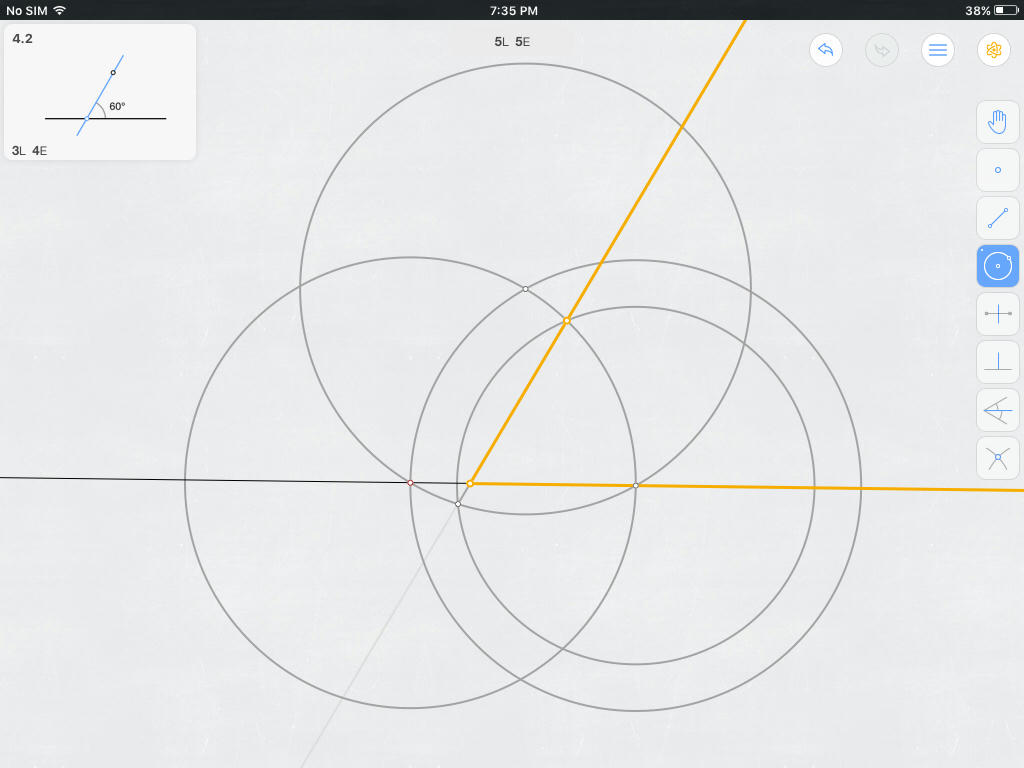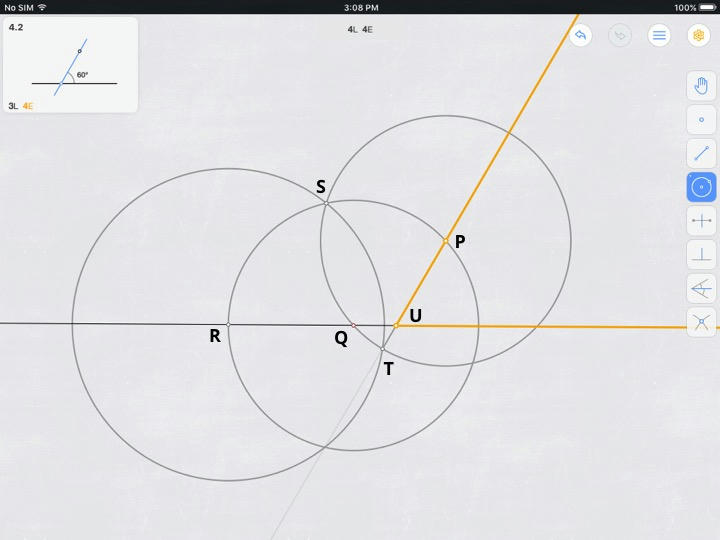# Construct 60° angle through point, other line in only four compass-and-straightedge steps

PROBLEM Here is a surprisingly intriguing challenge posed on Euclidea, a mobile app for Euclidean constructions: Construct a 60° angle through both a point $P$ and an external (infinite) line $\mathscr{l}$ using:

1. Only three lines at most, OR
2. Only four elementary Euclidean constructions at most.

HINTS FROM EUCLIDEA The problem is not solved until the line from $P$ to the appropriate point in $\mathscr{l}$ is fully constructed. Also, the variants can be solved by the following ordered sequences of moves:

1. Variant 1: Circle, circle, perpendicular bisector.
2. Variant 2: Circle, circle, circle, line.

DEFINITIONS Only unmarked straightedges and collapsing compasses may be used. The following table shows how moves are scored.

1. Construct a point: 0 lines (L), 0 elementary Euclidean moves (E).
2. Marking the intersection of two curves with a point: 0L, 0E.
3. Construct a new line (segment): 1L, 1E.
4. Construct an old line (segment): 1L, 1E.
5. Construct a circle (non-collapsible compass): 1L, 1E.
6. Construct the perpendicular bisector of a line segment: 1L, 3E.
7. Construct a new line perpendicular to an old line: 1L, 3E.
8. Construct an angle bisector: 1L, 4E.

RESEARCH Constructing a 60° angle involving only $\mathscr{l}$ is well-attested. Unfortunately, there are no questions on Mathematics Stackexchange about the $P$-constrained versions above. A Google search does not reveal anything promising.

BEST ATTEMPT YET The following figure accompanies the construction below, where the given and goal are shown as an inset:1. Construct $\bigcirc{A}$ centered on a point $A$ (in red) in $\mathscr{l}$ and with radius $AP$ [1L, 1E running total].
2. Let $B$ be the rightmost point where $\bigcirc{A}$ $\cap$ $\mathscr{l}$ [1L, 1E running total].
3. Construct $\bigcirc{B_{big}}$ centered on $B$ and with radius $AB$ [2L, 2E running total].
4. Construct $\bigcirc{B_{small}}$ centered on $B$ and with radius $AP$ [3L, 3E running total].
5. Let $C$ be the uppermost point where $\bigcirc{A}$ $\cap$ $\bigcirc{B_{big}}$ [3L, 3E running total].
6. Construct $\bigcirc{C}$ centered on $C$ and with radius $AC$; $m\angle{ABC} = 60°$ [4L, 4E running total].
7. Let $D$ be the leftmost point where $\bigcirc{C}$ $\cap$ $\bigcirc{B_{small}}$ [4L, 4E running total].
8. Construct $\overline{DP}$ [5L, 5E running total].
9. Let $E$ be the point where $\overline{DP}$ $\cap$ $\mathscr{l}$. $m\angle{PEB} = 60°$, which was what we wanted. [5L, 5E running total].
• Well, make a circle centered at P that crosses the line. From one of the intersections, draw a circle with same radios (I assume you can keep it). Connect P to the original intersection and the new created by the second circle - the angel between them $60^0$
– Moti
Mar 12 '16 at 4:03
• @Moti, thanks for your thoughts. While your solution does produce a 60° angle, this 60° angle is not through (i.e., does not involve) both $P$ and 𝓁, but rather only $P$. It therefore does not satisfy the constraints in the problem. Any suggestions?
– PDE
Mar 12 '16 at 4:37
• It depends on the radius of the first circle that could be large enough to intersect with l
– Moti
Mar 13 '16 at 6:33
• I'm playing the game and enjoying it quite a lot, but I don't know how you got the hints. This is a little off topic but can you show how? Sep 29 '16 at 10:31
• @nullgraph The iPad version certainly has hints. Perhaps the desktoo one has, too.
– PDE
Sep 30 '16 at 12:19

### Variant 1

@Blue has provided a solution, excavated here from the depths of the discussions above:1. Construct a point $Q \in$ the given external line $\ell$ [0L, 0E].
2. Construct $\bigcirc P$ with radius $PQ$ [1L, 1E].
• Let point $Q' := \bigcirc P \cap \ell \neq Q$.
3. Construct $\bigcirc Q$ with radius $PQ$ [2L, 2E].
• Let points $S, S' := \bigcirc P \cap \bigcirc Q$; S is the 'upper' intersection WLOG.
• $m\angle PQS = 60° \implies m\overset{\mmlToken{mo}{⏜}}{PS\,} = 60°$.
4. Construct $\overline{Q'S'}$ [3L, 3E].
• Resulting $\angle QQ'S'$ inscribes $\overset{\mmlToken{mo}{⏜}}{PS\,} \implies m\angle QQ'S' = 30°$
5. Construct $\ell'$, the perpendicular bisector of $\overline{Q'S'}$ [4L, 6E].
• Let $U := \ell' \cap \ell$.
• Let $V := \ell' \cap \overline{Q'S'}$.
• By construction, $\angle UVQ'$ is a right angle.
• Therefore, $m\angle VUQ' = 60°$.
• Moreover, $\ell'$ perpendicularly bisects a chord $\overline{Q'S'}$ of a circle $\bigcirc P \implies \ell'$ contains a diameter of $\bigcirc P \implies \ell'$ passes through $P$.
• By construction, $\angle VUQ' = \angle PUQ = 60°$, QEF.

Also, Euclidea gives additional points for constructing all possible versions of each geometric object in question. In this case, two possible angles can be produced. Below for completeness, therefore, is the Variant 1-type construction that produces both angles.### Variant 2

Variant 2 has been solved by @Blue above. A simple angle-chasing proof of that construction is also above. For what it's worth, though, here is an analytical proof:1. Set $PQ = radius(\bigcirc P) = radius(\bigcirc Q) := 1$.
• $m\angle PRS = 60°$
2. Define a right-handed coordinate system.
• $Q := (0, 0)$.
• $R := (-1, 0)$.
3. Let $\theta := 2 m\angle PQU$.
• $P = (cos(2\theta), sin(2\theta))$.
• $S = (cos(2\theta + 60°), sin(2\theta + 60°))$.
4. Calculate $RS^2 = radius^2(\bigcirc R)$. \begin{align} RS^2 & = (x_S - x_R)^2 + (y_S - y_R)^2 \\ & = (cos(2\theta + 60°) - (-1))^2 + (sin(2\theta + 60°) - (0))^2 \\ & = (cos^2(2\theta + 60°) + 2cos(2\theta + 60°) + 1) + sin^2(2\theta + 60°) \\ & = 2 + 2cos(2\theta + 60°) ) \\ RS^2 & = 4cos^2(\theta + 30°) \\ \end{align}
5. Determine the equation of $\bigcirc R$. \begin{align} RS^2 & = (x - x_R)^2 + (y - y_R)^2 \\ 4cos^2(\theta + 30°) & = (x + 1)^2 + y^2 \\ \end{align}
6. Determine the equation of $\bigcirc P$. \begin{align} PQ^2 & = (x - x_P)^2 + (y - y_P)^2 \\ 1 & = (x - cos(2\theta))^2 + (y - sin(2\theta))^2 \\ \end{align}
7. Find the coordinates of $T$, the other intersection of $\bigcirc P$ and $\bigcirc R$.
\left\{ \begin{aligned} 4cos^2(\theta + 30°) & = (x_T + 1)^2 + y_T^2 \\ 1 & = (x_T - cos(2\theta))^2 + (y_T - sin(2\theta))^2 \\ \end{aligned} \right. WolframAlpha solves this system as $T := \bigcirc P \cap \bigcirc R = \left(cos(2\theta) - \frac{1}{2}, sin(2\theta) - \frac{\sqrt{3}}{2} \right)$.
8. Find the slope $s$ of $\overline{PT}$. \begin{align} s & = \frac{y_T - y_P}{x_T - x_P} = \frac{\left(sin(2\theta) - \frac{\sqrt{3}}{2}\right) - sin(2\theta)}{\left(cos(2\theta) - \frac{1}{2}\right) - cos(2\theta)} \\ s & = \sqrt{3} \end{align}
9. The angle of $\overline{PT}$ with respect to the positive x-axis is $atan(s) = 60°$. QED. $\square$Let $Q$ be a point on the given line.

1. Construct $\bigcirc P$ through $Q$. [$1$L, $1$E]
2. Construct $\bigcirc Q$ through $P$. [$2$L, $2$E]

• Let $\bigcirc Q$ meet the given line at $R$.
• Let $\bigcirc Q$ meet $\bigcirc P$ at $S$. (Either intersection works.)
3. Construct $\bigcirc R$ through $S$. [$3$L, $3$E]

• Let $\bigcirc R$ meet $\bigcirc P$ at $T \neq S$.
4. Construct $\overleftrightarrow{PT}$. [$4$L, $4$E]

• Let $\overleftrightarrow{PT}$ meet the given line at $U$.
• Observe: $\angle PUQ = 60^\circ$. $\square$

Here's proof of the construction.We take $\bigcirc P$ through $Q$ and $\bigcirc Q$ through $P$, with the circles meeting at $S$ and $S^\prime$. (Note that $\triangle PQS^\prime$ is equilateral.) Let $R$ be any point on major arc $\stackrel{\frown}{SS^\prime}$ of $\bigcirc Q$, and extend $\overline{QR}$ to meet $\bigcirc P$ at $Q^\prime$. Further, extend $\overline{RS^\prime}$ to meet $\bigcirc P$ at $T$, and let $\overleftrightarrow{PT}$ meet $\overline{RQ^\prime}$ at $U$. (Without too much trouble, one can show that $\overline{RS} \cong \overline{RT}$, so that the $T$ here agrees with the point described in my above answer; or, one can use the current description as a "circle, circle, line, line" solution variant.)

From here, it's a very short angle chase. (Thanks to @PDE for greatly simplifying my unnecessarily-elaborate first attempt.) Simply assign $\angle R = \theta$, and note that $\triangle QRS^\prime$ and $\triangle PTS^\prime$ are isosceles to get $\angle T = 120^\circ - \theta$. Then $\angle U$ of $\triangle RTU$ must be $60^\circ$, as claimed. $\square$

(A slightly-modified angle chase shows, if we let $\overline{RS}$ meet $\bigcirc P$ at $T^\prime$, then $\overline{PT^\prime}$ meets $\overline{RQ^\prime}$ at a $60^\circ$ angle, as well.)

Incidentally, I originally solved the problem by trial and error, based on the "circle, circle, circle, line" hint provided: The only way to make the first circle was to introduce a new point, which had to lie on the line; easy. This gave me three points to use, but really only two distinguishable second circles to make. Each choice provided a few more points to determine a third circle, but I knew that the third circle had to produce points with which I could make the $60^\circ$ angle with the original line; it took a few tries before I arrived at the proper combination. I verified the construction with an ugly coordinate proof, and then eventually hit upon the angle chase shown above.

• Thanks! This certainly answers one variant of the question (the 4E variant, i.e., variant 2). Just FYI: I've corrected the scoring since Euclidea counts this solution as a [4L, 4E] solution. Very counterintuitively from the view of most people with a geometry background, the app scores each circle as a [1L, 1E] move.
– PDE
Mar 12 '16 at 13:58
• @PDE: Scoring circle-moves as line-moves makes sense (lines "are" circles, from an appropriate point of view); I simply didn't pay proper attention to that aspect of your description of the scoring. Fixed (since apparently your attempted edits were rejected by someone).
– Blue
Mar 12 '16 at 14:58
• What's more interesting to me now is proving why this construction works. I've been able to do so by analytic geometry (set the radius of $\bigcirc{P}$ = radius of $\bigcirc{Q}$ = 1, let $\mathscr{l}$ be the x-axis, let $Q$ be the origin, find the equation of $\bigcirc{R}$, find the coordinates of $T$, calculate the arctangent of the slope of $\overline{PT}$). But that approach is not very satisfying. Any classically-oriented hints on why your approach works?
– PDE
Mar 12 '16 at 16:17
• An even faster angle chase: $$m\angle TRU = m\angle RS'Q := \theta \implies m\angle TS'P = 120° - \theta \implies m\angle S'TP := m\angle RTU = 120° - \theta$$ Therefore, $$m\angle RUT = 180° - m\angle TRU - m\angle RTU = 180° - \theta - (120° - \theta) = 60°$$ QED.
– PDE
Mar 13 '16 at 14:13
• @PDE: By the way ... For Variant 1, note that $\overleftrightarrow{PT}$ could've been constructed as the perpendicular bisector of $\overline{SQ^\prime}$ in my second figure. (This is easily proven, since $\angle QQ^\prime S = 30^\circ$, as an inscribed angle subtending an arc of measure $60^\circ$.)
– Blue
Mar 13 '16 at 15:14

1.- Draw circle using any point on the infinite line as center and the initial point as radius.

2.- Draw another circle using the initial point as center and the center of the first cicle as radius.

3.- Draw a line from initial point to 2 circles intersection.